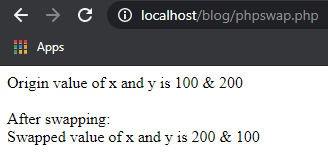# PHP program to swap two variables without a 3rd variableIn this post, let’s write a simple PHP snippet to Swap two variables without using a temporary variable. Basically, we are going to declare two variables x and y and then swap the values of x and y without introducing a 3rd variable.

```';

print 'After swapping: ';

//now swap the values without introducing a 3rd variable
list(\$x, \$y) = array(\$y, \$x);

print 'Swapped value of x and y is ' . \$x . ' & ' . \$y;

?>```

Sample output of the above program is here,The post PHP program to swap two variables without a 3rd variable appeared first on TutorialsMade.